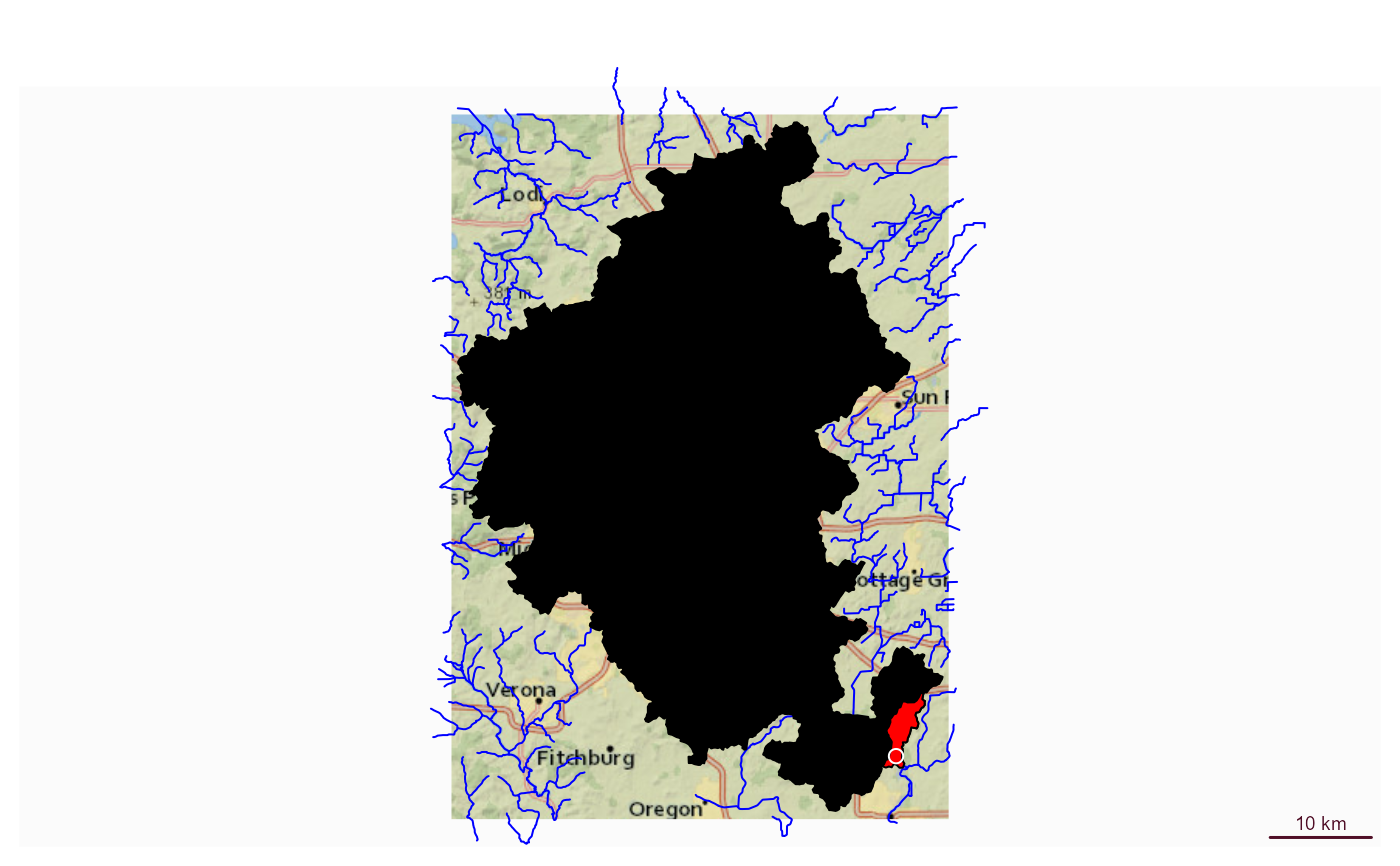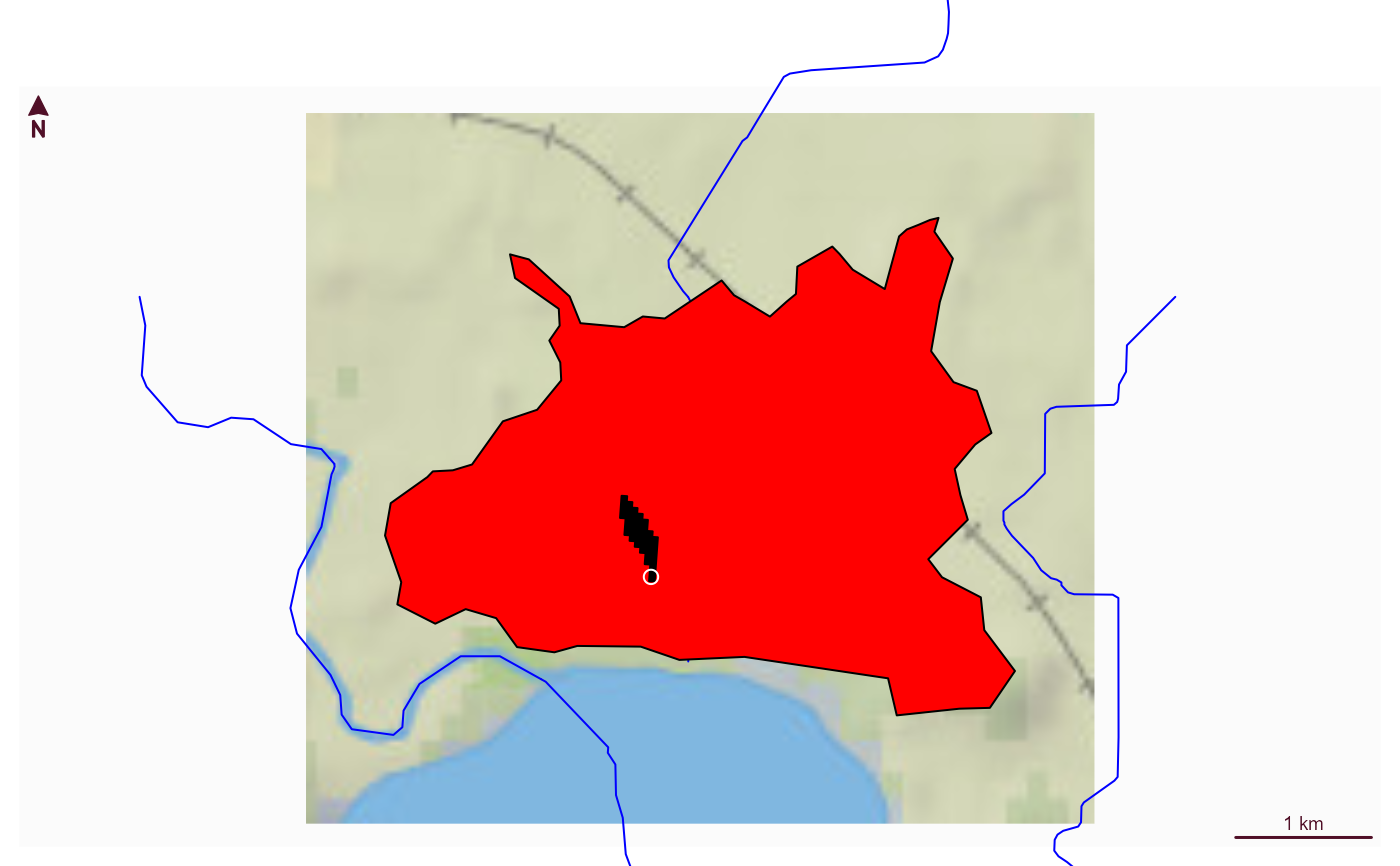Uses catchment splitting web service to retrieve the portion of a catchment upstream of the point provided.

get_split_catchment(point, upstream = TRUE)

## Arguments

point

scf POINT including crs as created by: sf::st_sfc(sf::st_point(.. ,..), crs)

upstream

logical If TRUE, the entire drainage basin upstream of the point provided is returned in addition to the local catchment.

## Value

sf data.frame containing the local catchment, the split portion and optionally the total dranage basin.

## Examples

# \donttest{
point <- sf::st_sfc(sf::st_point(x = c(-89.2158, 42.9561)), crs = 4326)

trace <- get_raindrop_trace(point)

if(inherits(trace, "sf")) {

(snap_point <- sf::st_sfc(sf::st_point(trace\$intersection_point[]),
crs = 4326))

(catchment <- get_split_catchment(snap_point))

bbox <- sf::st_bbox(catchment) + c(-0.005, -0.005, 0.005, 0.005)

nhdplusTools::plot_nhdplus(bbox = bbox, cache_data = FALSE)

plot(sf::st_transform(sf::st_geometry(catchment), 3857), add = TRUE, col = "black")
plot(sf::st_transform(sf::st_geometry(catchment), 3857), add = TRUE, col = "red")
plot(sf::st_transform(sf::st_sfc(point, crs = 4326), 3857), add = TRUE, col = "white")

(catchment <- get_split_catchment(snap_point, upstream = FALSE))

bbox <- sf::st_bbox(catchment) + c(-0.005, -0.005, 0.005, 0.005)

nhdplusTools::plot_nhdplus(bbox = bbox, cache_data = FALSE)

plot(sf::st_transform(sf::st_geometry(catchment), 3857), add = TRUE, col = "red")
plot(sf::st_transform(sf::st_geometry(catchment), 3857), add = TRUE, col = "black")
plot(sf::st_transform(sf::st_sfc(point, crs = 4326), 3857), add = TRUE, col = "white")

pour_point <- sf::st_sfc(sf::st_point(x = c(-89.25619, 42.98646)), crs = 4326)

(catchment <- get_split_catchment(pour_point, upstream = FALSE))

bbox <- sf::st_bbox(catchment) + c(-0.005, -0.005, 0.005, 0.005)

nhdplusTools::plot_nhdplus(bbox = bbox, cache_data = FALSE)

plot(sf::st_transform(sf::st_geometry(catchment), 3857), add = TRUE, col = "red")
plot(sf::st_transform(sf::st_geometry(catchment), 3857), add = TRUE, col = "black")
plot(sf::st_transform(sf::st_sfc(pour_point, crs = 4326), 3857), add = TRUE, col = "white")
}
#> Spherical geometry (s2) switched off
#> Found invalid geometry, attempting to fix.
#> although coordinates are longitude/latitude, st_intersects assumes that they
#> are planar
#> Spherical geometry (s2) switched on
#> Spherical geometry (s2) switched off
#> although coordinates are longitude/latitude, st_intersects assumes that they
#> are planar
#> Spherical geometry (s2) switched on
#> Spherical geometry (s2) switched off
#> although coordinates are longitude/latitude, st_intersects assumes that they
#> are planar
#> Spherical geometry (s2) switched on
#> Spherical geometry (s2) switched off
#> although coordinates are longitude/latitude, st_intersects assumes that they
#> are planar
#> Spherical geometry (s2) switched on
#> Spherical geometry (s2) switched off
#> although coordinates are longitude/latitude, st_intersects assumes that they
#> are planar
#> Spherical geometry (s2) switched on
#> Zoom set to: 10
#> Spherical geometry (s2) switched off
#> although coordinates are longitude/latitude, st_intersects assumes that they
#> are planar
#> Spherical geometry (s2) switched on
#> Spherical geometry (s2) switched off
#> although coordinates are longitude/latitude, st_intersects assumes that they
#> are planar
#> Spherical geometry (s2) switched on
#> Spherical geometry (s2) switched off
#> although coordinates are longitude/latitude, st_intersects assumes that they
#> are planar
#> Spherical geometry (s2) switched on
#> Spherical geometry (s2) switched off
#> although coordinates are longitude/latitude, st_intersects assumes that they
#> are planar
#> Spherical geometry (s2) switched on
#> Spherical geometry (s2) switched off
#> although coordinates are longitude/latitude, st_intersects assumes that they
#> are planar
#> Spherical geometry (s2) switched on
#> Zoom set to: 12#> Spherical geometry (s2) switched off
#> although coordinates are longitude/latitude, st_intersects assumes that they
#> are planar
#> Spherical geometry (s2) switched on
#> Spherical geometry (s2) switched off
#> although coordinates are longitude/latitude, st_intersects assumes that they
#> are planar
#> Spherical geometry (s2) switched on
#> Spherical geometry (s2) switched off
#> although coordinates are longitude/latitude, st_intersects assumes that they
#> are planar
#> Spherical geometry (s2) switched on
#> Spherical geometry (s2) switched off
#> although coordinates are longitude/latitude, st_intersects assumes that they
#> are planar
#> Spherical geometry (s2) switched on
#> Spherical geometry (s2) switched off
#> Warning: No nonnetwork features found
#> Spherical geometry (s2) switched on
#> Zoom set to: 12# }